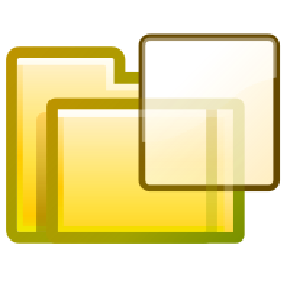geoffrey
/
dotfiles
Public part of my dotfiles.
You can not select more than 25 topics Topics must start with a letter or number, can include dashes ('-') and can be up to 35 characters long.

#### 21 lines 448 B Raw Blame History

 `#!/usr/bin/env python3` ``` ``` `import sys` `import random` ``` ``` `for line in sys.stdin:` ` nl = ""` ` word = ""` ` for c in line:` ` if c.isalpha():` ` word += c` ` else:` ` if len(word) > 3:` ` wrd = list(word)[1:-1]` ` random.shuffle(wrd)` ` nl += word + "".join(wrd) + word[-1]` ` else:` ` nl += word` ` word = ""` ` nl += c` ``` print(nl, end="") ```